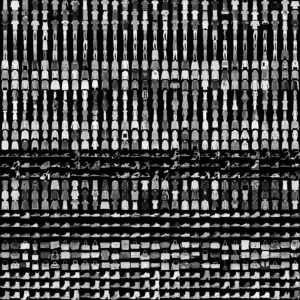Introducing Lit-LLaMA: a minimal, optimized rewrite of LLaMA licensed under Apache 2.0 →

# Unit 4 Exercises

## Exercise 1: Changing the Number of Layers

In this exercise, we are toying around with the multilayer perceptron architecture from Unit 4.3.

In Unit 4.3, we fit the following multilayer perceptron on the MNIST dataset:

class PyTorchMLP(torch.nn.Module):
def __init__(self, num_features, num_classes):
super().__init__()

self.all_layers = torch.nn.Sequential(
# 1st hidden layer
torch.nn.Linear(num_features, 50),
torch.nn.ReLU(),
# 2nd hidden layer
torch.nn.Linear(50, 25),
torch.nn.ReLU(),
# output layer
torch.nn.Linear(25, num_classes),
)

def forward(self, x):
x = torch.flatten(x, start_dim=1)
logits = self.all_layers(x)
return logits

This network had 40,785 parameters (see Quiz on how to calculate this number), and it achieved the following accuracy values:

• Train Acc 97.24%
• Val Acc 95.64%
• Test Acc 96.46%

Can you change the architecture to achieve the same (or better) performance with fewer parameters and only 1 hidden layer?

PS: You may also try to add additional layers, but as a rule of thumb, using more than two hidden layers in a multi-layer perceptron rarely improves the predictive performance.

You can use th notebook in this folder as a template: Unit 4 exercise 1

## Exercise 2: Implementing a Custom Dataset Class for Fashion MNIST

In this exercise, we are going to train the multilayer perceptron from Unit 4.3 on a new dataset, Fashion MNIST, based on the PyTorch Dataset concepts introduced in Unit 4.4.

Fashion MNIST is a dataset with a same number of images and image dimension as MNIST. However, instead of handwritten digits, it contains low-resolution images of fashion objects.Since the image format of MNIST and Fashion MNIST is identical, we can use the multilayer perceptron code from Unit 4.3 without any modification. The only adjustments we have to make is replacing the MNIST dataset code with a custom Dataset class for Fashion MNIST.

To get started, download the GitHub folder https://github.com/zalandoresearch/fashion-mnist and place the data subfolder next to the notebook. Then, implement the custom Dataset class and train the multilayer perceptron. You should get at least >85% training accuracy.

Hint: You may use the following Dataset code as a starter and fill out the missing blanks:

class MyDataset(Dataset):
def __init__(self, ..., transform=None):

self.transform = transform
# ...

def __getitem__(self, index):
# ...
img = torch.tensor(img).to(torch.float32)
img = img/255.
# ...

if self.transform is not None:
img = self.transform(img)

# ...

def __len__(self):
return self.labels.shape

You can use th notebook in this folder as a template: Unit 4 exercise 2

PS: If you get stuck, please don’t hesitate to reach out for help via the forum!